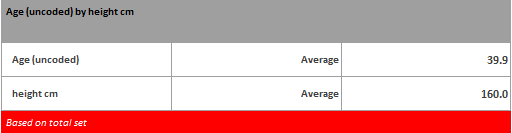Usually averages are calculated on Scale variables (i.e. age, height, etc.) but Datadynamic allow users to calculate averages also for categorical and calculated variables.

To explain how to produce a table containing only averages, let's start with an example like the table reported belowIn this table, we've put together 2 variables (Age and Height) down the side and just kept the average.

Practically what you need to do is:

+ drag and drop the variables you want to calculate the averages down the side

+ click on 'Table Options' and make sure that row/column totals are un-checked and 'column averages' is checked. See screenshot below+ always in 'Table Options', but in 'Formatting options' tab, make sure that both 'Merge into one table' and 'Hide categories rows' are checked (see screenshot below). This needs to be done a) to produce one unique table instead of 2 separate (in this example) and b) to hide all categories, avoiding the table to contain all the possible values the variables can assume.You can follow similar steps as described above by checking the 2 videos on our Youtube channel: